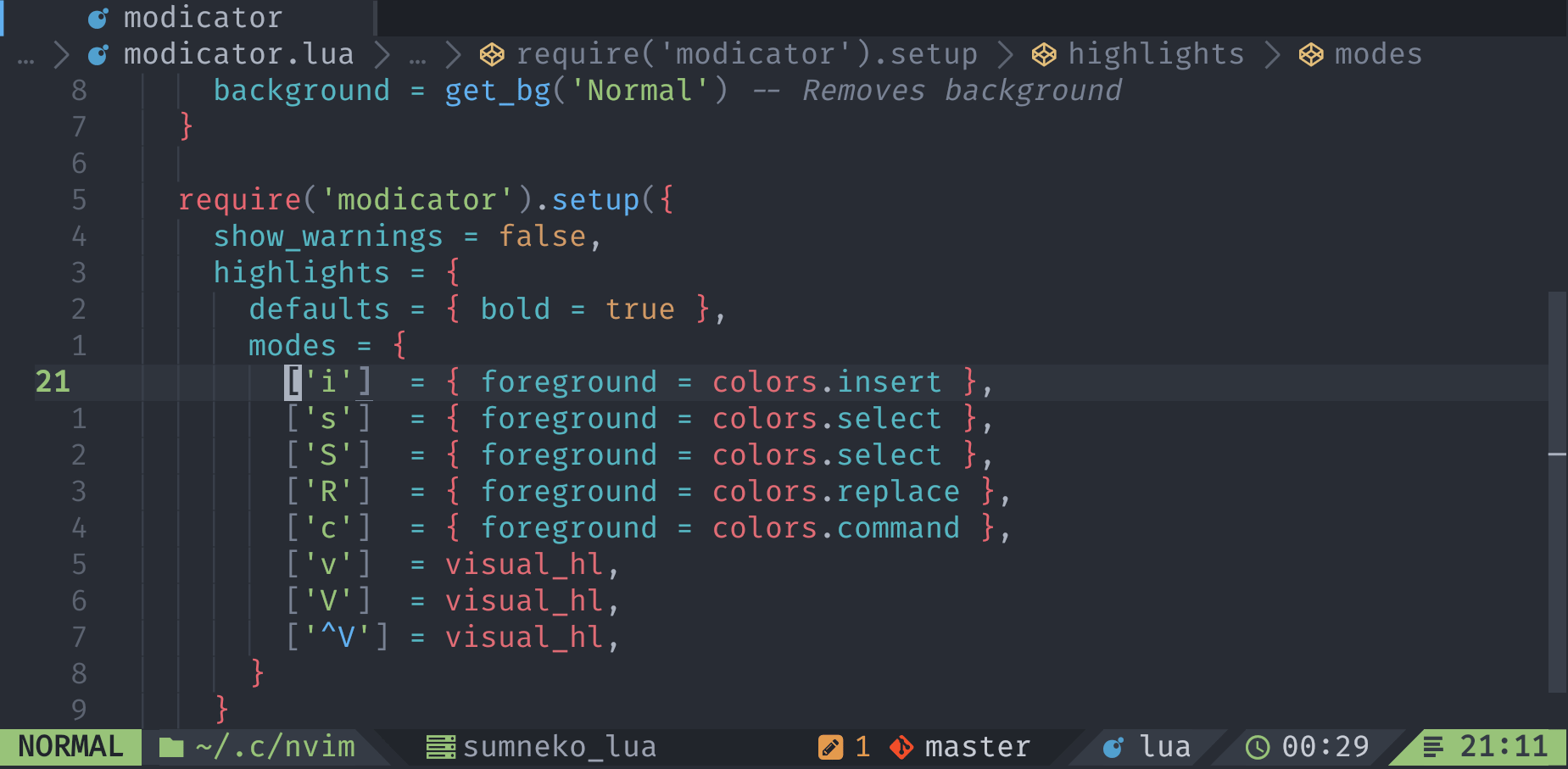## mawkler/modicator.nvimgithub
pluginbars-and-linescursorline101014

# 💡 Modicator.nvim

Cursor line number mode indicator.

A small Neovim plugin that changes the foreground color of the `CursorLineNr` highlight based on the current Vim mode.## Setup

``````require('modicator').setup()
``````

Note that modicator requires you to have `termguicolors`, `cursorline`, `number` set. In Lua this is done by adding

``````vim.o.termguicolors = true
vim.o.cursorline = true
vim.o.number = true
``````

Modicator sets the Normal mode highlight foreground based on the default foreground color of `CursorLineNr` so if you're using a colorscheme make sure that it gets loaded before this plugin.

With lazy.nvim:

``````return {
'mawkler/modicator.nvim',
init = function()
-- These are required for Modicator to work
vim.o.cursorline = true
vim.o.number = true
vim.o.termguicolors = true
end,
config = function()
require('modicator').setup({
-- ...
})
end,
}
``````

Or with packer.nvim:

``````use {
'mawkler/modicator.nvim',
setup = function()
-- These are required for Modicator to work
vim.o.cursorline = true
vim.o.number = true
vim.o.termguicolors = true
end,
config = function()
require('modicator').setup({
-- ...
})
end
}
``````

## Configuration

Use `highlights.modes[<mode>]` to set the color for each mode, and pass it to `require('modicator').setup()`, as seen below. Each mode in `highlights.modes` can have a `foreground`, `background`, `bold` and `italic` entry. The key for each mode is the output `mode()` for that mode. Check out `:help mode()` for more information.

For normal mode, Modicator uses the `CursorLineNr`'s `fg` highlight.

Default configuration:

``````local modicator = require('modicator')

-- NOTE: Modicator requires line_numbers and cursorline to be enabled
modicator.setup({
-- Show warning if any required option is missing
show_warnings = true,
highlights = {
-- Default options for bold/italic. You can override these individually
-- for each mode if you'd like as seen below.
defaults = {
foreground = modicator.get_highlight_fg('CursorLineNr'),
background = modicator.get_highlight_bg('CursorLineNr'),
bold = false,
italic = false
},
-- Color and bold/italic options for each mode. You can add a bold and/or
-- italic key pair to override the default highlight for a specific mode if
-- you would like.
modes = {
['n'] = {
foreground = modicator.get_highlight_fg('CursorLineNr'),
},
['i']  = {
foreground = modicator.get_highlight_fg('Question'),
},
['v']  = {
foreground = modicator.get_highlight_fg('Type'),
},
['V']  = {
foreground = modicator.get_highlight_fg('Type'),
},
[''] = { -- This symbol is the ^V character
foreground = modicator.get_highlight_fg('Type'),
},
['s']  = {
foreground = modicator.get_highlight_fg('Keyword'),
},
['S']  = {
foreground = modicator.get_highlight_fg('Keyword'),
},
['R']  = {
foreground = modicator.get_highlight_fg('Title'),
},
['c']  = {
foreground = modicator.get_highlight_fg('Constant'),
},
},
},
})
``````